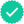Question

Find the charge q(t) flowing through an device if the current is:
(c) i(t)= 20 cos(10t + π/6)10^-6A, q(0)=2(10^-6)C

My problem in this question is the substitution.
I know that u=10t+π\6 , and u'=10, but the u' doesn't equal to the 20 in front of the cos, how do I find the derivative that will equal the "20".

Expert SolutionStudents who’ve seen this question also like:Your question is solved by a Subject Matter ExpertWant to see this answer and more?
Experts are waiting 24/7 to provide step-by-step solutions in as fast as 30 minutes!*
*Response times may vary by subject and question complexity. Median response time is 34 minutes for paid subscribers and may be longer for promotional offers.8+ million solutions